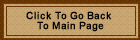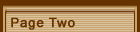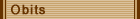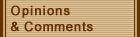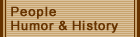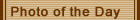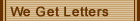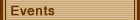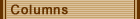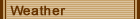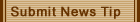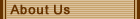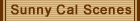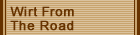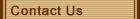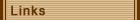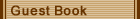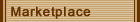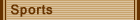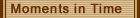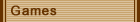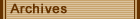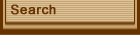## Math Brainteaser from 1907

(09/29/2001)

Transcribed by Norma Knotts Shaffer from microfilm of the Calhoun Chronicle dated 4/20/1907.

How Many Eggs Did Jones Buy

Godfrey L. Cabot of Boston, Mass., has worked out a solution for the problem that recently bothered Jones and has also bothered several of our subscribers.  The problem was like this:

Jones asked a Grantsville grocer the price of eggs and ordered 24 cents worth.  The grocer replied that two more eggs than he was then selling for 24 cents would make them two cents less a dozen than they then were.  Jones didn't know how many eggs he should have received, and in fact, neither did we, until Mr. Cabot came to our relief in this manner.

How many eggs did Jones buy?

Let X equal number of eggs Jones bought, and Y equal number of cents per egg.

(1) xy equals 24

(2.) (X plus 2) x (Y less 1-6) equals 24; or,

(3) XY less Xover6 plus 2Y less 1-3 equals 24.

As XY equals 24, 2Y less Xover6 less 1-3 equals 0, and 2Y equals Xover6 plus 1-3

XY equals X {X plus 2over12} equals 24.  Y equals X plus 2over12

X squared plus 2x equals 288.

X squared  plus 1 equals 289

X squared plus 2x plus 1 equals 289

X squared  plus 2x plus 1 equals {x plus 1}squared. x plus 1 equals the square root of 289 equals 17. x equals 16 Q.E.D.

Therefore Jones was paying 18 cents per dozen for eggs and if he could have bought them at 16 cents a doz. he would have bought two more eggs with his 24 cents.

Godfrey L. Cabot.